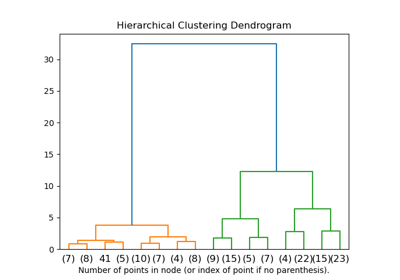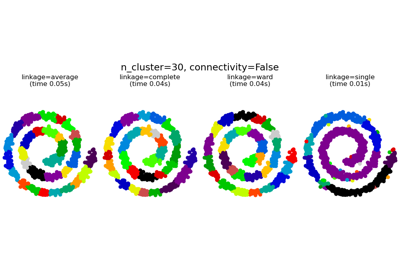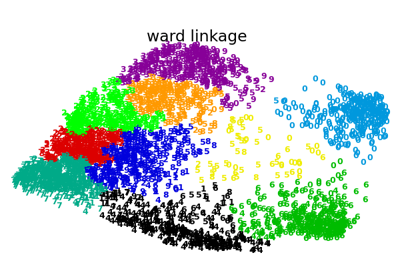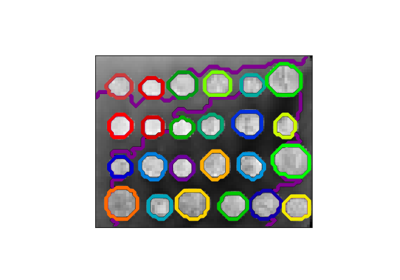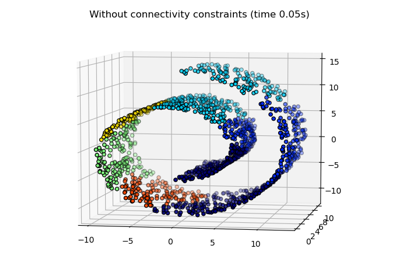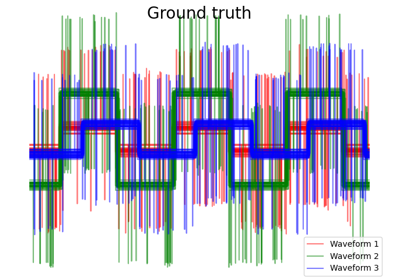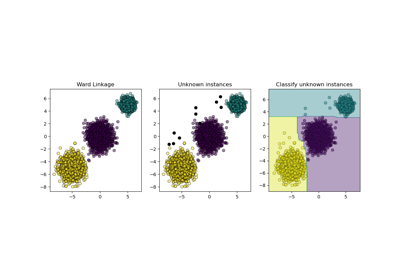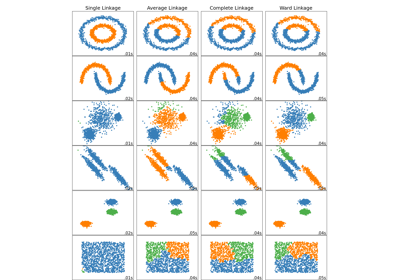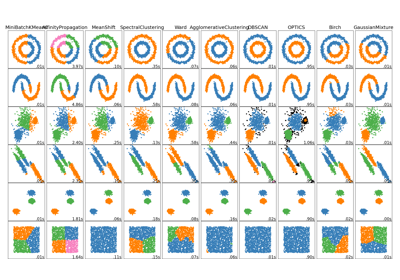# sklearn.cluster.AgglomerativeClustering¶

class sklearn.cluster.AgglomerativeClustering(n_clusters=2, *, affinity='euclidean', memory=None, connectivity=None, compute_full_tree='auto', linkage='ward', distance_threshold=None)[source]

Agglomerative Clustering

Recursively merges the pair of clusters that minimally increases a given linkage distance.

Read more in the User Guide.

Parameters
n_clustersint or None, default=2

The number of clusters to find. It must be None if distance_threshold is not None.

affinitystr or callable, default=’euclidean’

Metric used to compute the linkage. Can be “euclidean”, “l1”, “l2”, “manhattan”, “cosine”, or “precomputed”. If linkage is “ward”, only “euclidean” is accepted. If “precomputed”, a distance matrix (instead of a similarity matrix) is needed as input for the fit method.

memorystr or object with the joblib.Memory interface, default=None

Used to cache the output of the computation of the tree. By default, no caching is done. If a string is given, it is the path to the caching directory.

connectivityarray-like or callable, default=None

Connectivity matrix. Defines for each sample the neighboring samples following a given structure of the data. This can be a connectivity matrix itself or a callable that transforms the data into a connectivity matrix, such as derived from kneighbors_graph. Default is None, i.e, the hierarchical clustering algorithm is unstructured.

compute_full_tree‘auto’ or bool, default=’auto’

Stop early the construction of the tree at n_clusters. This is useful to decrease computation time if the number of clusters is not small compared to the number of samples. This option is useful only when specifying a connectivity matrix. Note also that when varying the number of clusters and using caching, it may be advantageous to compute the full tree. It must be True if distance_threshold is not None. By default compute_full_tree is “auto”, which is equivalent to True when distance_threshold is not None or that n_clusters is inferior to the maximum between 100 or 0.02 * n_samples. Otherwise, “auto” is equivalent to False.

Which linkage criterion to use. The linkage criterion determines which distance to use between sets of observation. The algorithm will merge the pairs of cluster that minimize this criterion.

• ward minimizes the variance of the clusters being merged.

• average uses the average of the distances of each observation of the two sets.

• complete or maximum linkage uses the maximum distances between all observations of the two sets.

• single uses the minimum of the distances between all observations of the two sets.

New in version 0.20: Added the ‘single’ option

distance_thresholdfloat, default=None

The linkage distance threshold above which, clusters will not be merged. If not None, n_clusters must be None and compute_full_tree must be True.

New in version 0.21.

Attributes
n_clusters_int

The number of clusters found by the algorithm. If distance_threshold=None, it will be equal to the given n_clusters.

labels_ndarray of shape (n_samples)

cluster labels for each point

n_leaves_int

Number of leaves in the hierarchical tree.

n_connected_components_int

The estimated number of connected components in the graph.

New in version 0.21: n_connected_components_ was added to replace n_components_.

children_array-like of shape (n_samples-1, 2)

The children of each non-leaf node. Values less than n_samples correspond to leaves of the tree which are the original samples. A node i greater than or equal to n_samples is a non-leaf node and has children children_[i - n_samples]. Alternatively at the i-th iteration, children[i] and children[i] are merged to form node n_samples + i

Examples

>>> from sklearn.cluster import AgglomerativeClustering
>>> import numpy as np
>>> X = np.array([[1, 2], [1, 4], [1, 0],
...               [4, 2], [4, 4], [4, 0]])
>>> clustering = AgglomerativeClustering().fit(X)
>>> clustering
AgglomerativeClustering()
>>> clustering.labels_
array([1, 1, 1, 0, 0, 0])


Methods

 fit(X[, y]) Fit the hierarchical clustering from features, or distance matrix. fit_predict(X[, y]) Fit the hierarchical clustering from features or distance matrix, and return cluster labels. get_params([deep]) Get parameters for this estimator. set_params(**params) Set the parameters of this estimator.
__init__(n_clusters=2, *, affinity='euclidean', memory=None, connectivity=None, compute_full_tree='auto', linkage='ward', distance_threshold=None)[source]

Initialize self. See help(type(self)) for accurate signature.

fit(X, y=None)[source]

Fit the hierarchical clustering from features, or distance matrix.

Parameters
Xarray-like, shape (n_samples, n_features) or (n_samples, n_samples)

Training instances to cluster, or distances between instances if affinity='precomputed'.

yIgnored

Not used, present here for API consistency by convention.

Returns
self
fit_predict(X, y=None)[source]

Fit the hierarchical clustering from features or distance matrix, and return cluster labels.

Parameters
Xarray-like, shape (n_samples, n_features) or (n_samples, n_samples)

Training instances to cluster, or distances between instances if affinity='precomputed'.

yIgnored

Not used, present here for API consistency by convention.

Returns
labelsndarray, shape (n_samples,)

Cluster labels.

get_params(deep=True)[source]

Get parameters for this estimator.

Parameters
deepbool, default=True

If True, will return the parameters for this estimator and contained subobjects that are estimators.

Returns
paramsmapping of string to any

Parameter names mapped to their values.

set_params(**params)[source]

Set the parameters of this estimator.

The method works on simple estimators as well as on nested objects (such as pipelines). The latter have parameters of the form <component>__<parameter> so that it’s possible to update each component of a nested object.

Parameters
**paramsdict

Estimator parameters.

Returns
selfobject

Estimator instance.

## Examples using sklearn.cluster.AgglomerativeClustering¶